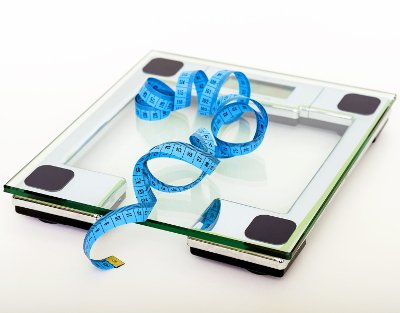# US Navy Body Fat Percentage Calculator

Share:

Enter your gender, age, waist circumference and neck circumference. Then click the Calculate Navy BFP button.

*
*
*
ft
Feet
in
Inches
cm
Centimeters
*
In
Inches
cm
Centimeters
*
in
Inches
cm
Centimeters
*
In
Inches
Cm
Centimeters

Similar Fitness Calculators

## About Navy Body Fat Percentage CalculatorThis calculator is used to calculate the body fat percentage based on USA's navy formula.

### Using Navy Body Fat Percentage calculator

1. Select the Gender.
2. select the Age.
3. Enter the Height either in feet/inches or centimeters.
4. Enter the Neck circumference either in inches or centimeters. (See guidelines below for taking measurement.)
5. Enter the Waist circumference either in inches or centimeters. (See guidelines below for taking measurement.)
6. Enter the Hip circumference if you have selected Female in gender field. (See guidelines below for taking measurement.)
7. Finally, click the Calculate Navy BFP button. The body fat percentage based on USA's navy formula will be calculated and the result will be displayed in the box below the button.

#### Guide For Measuring Neck Circumference

1. Use someone's help for measuring your neck circumference.
2. Be relaxed and look straight ahead.
3. Circumference of the neck should be measured at a point just below the larynx (Adam's apple).
4. Make sure shoulder muscles are not involved in the measurement

#### Guide For Measuring Waist Circumference

1. Wear little cloth as possible.
2. Stand straight with your feet close together.
3. Keep your arms down at the sides.
4. Take the measurement after the end of a normal expiration.
5. For Male: Measure the circumference of waist across the belly button. Repeat the measurements twice and take the average.
For Female: Measure the smallest circumference of the waist, (The smallest circumference is usually halfway between the lower point of sternum and the belly button) roughly one inch above the belly button. Repeat the measurements few times and take the smallest value.

#### Guide For Measuring Hip Circumference

1. Wear less cloth as possible.
2. Stand in front of a full body mirror.
3. Keep the feet close together with little space between them.
4. Place the tape around the maximum circumference of the buttocks. Make sure the tape is in horizontal position.
5. Breath normally. Take the measurement after the exhaust.

## Navy Body Fat Percentage (NBFP)

### Formula

In US Navy is using separate equations for men and women to calculate the percentage of body fat. These equations are formulated by James A. Hodgdon and Marcie B. Beckett.

#### Men

Density = -0.191 × Log10(waist - neck) + 0.155 × Log10(height) + 1.032

BF% = 100 × [(4.95 ÷ density) - 4.5]

#### Women

Density = -0.350 × Log10(waist + hip - neck) + 0.221 × Log10(height) + 1.296

BF% = 100 × [(4.95 ÷ density) - 4.5]

Where:

• waist = Waist Circumference in centimeters.
• hip = Hip Circumference in centimeters.
• neck = Neck Circumference in centimeters.
• height = Height in centimeters.

### Maximum Allowed US Navy Body Fat Limits

Here is the allowed limits of body fat percentage by US Navy. This table is based on the Navy Physical Readiness Program: Guide 4-Body Composition Assessment (BCA) (APR 2021).

Age (years) Men (BF%) Women (BF%)
17 to 21 22% 33%
22 to 29 23% 34%
30 to 39 24% 35%
40 and Above 26% 36%